﻿ 应用改进区块遗传算法求解置换流水车间调度问题
«上一篇文章快速检索 高级检索

 智能系统学报2019, Vol. 14Issue (3): 541-550  DOI: 10.11992/tis.2018010410

### 引用本文PEI Xiaobing, ZHANG Chunhua. An improved puzzle-based genetic algorithm for solving permutation flow-shop scheduling problems[J]. CAAI Transactions on Intelligent Systems, 2019, 14(3): 541-550. DOI: 10.11992/tis.201801041.### 文章历史

An improved puzzle-based genetic algorithm for solving permutation flow-shop scheduling problems
PEI Xiaobing , ZHANG ChunhuaSchool of Management, Tianjin University of Technology, Tianjin 300384, China
Abstract: Targeting the permutation flow-shop scheduling problem that minimizes maximum completion times, an improved block genetic algorithm combined with an ant colony algorithm is proposed. The algorithm uses a random mechanism and the improved opposition-based learning mechanism to generate the initial solution. It takes into account the diversity and quality of the initial population. Elite populations are generated through several generations of simple genetic algorithm operations. Based on the idea of using ant information density concentration’s statistical path and node information in the ant colony algorithm, the information carried by elite groups was counted. Position pheromone and dependent pheromone matrices were established. Mining blocks according to two matrices were developed and blocks were combined with non-blocks to form artificial chromosomes. Chromosomes were cut and recombined to increase chromosome quality. The binary race method was used to retain chromosomes with higher fitness. The algorithm was tested through the Reeves and Taillard instances, and the results were compared with other algorithms to verify effectiveness of the algorithm.
Key words: production scheduling    combinatorial optimization    genetic algorithms    ant colony optimization    building block    artificial chromosome

Chang等针对置换流水车间调度问题，提出一种基于区块的遗传算法(puzzle-based artificial chromosome genetic algorithm，p-ACGA)，将遗传算法和蚁群算法相结合，通过简单遗传算法的交叉、突变等操作产生精英群体，针对这些精英群体，利用蚁群算法的信息素浓度对工件与工件的关系进行统计分析，进而建立信息素相依矩阵并根据矩阵挖掘区块，利用区块和非区块重组形成人工染色体，然后将其注入到进化过程中。虽然p-ACGA能够避免局部最优问题，一定程度上改善了解的质量，加快了进化速度，但是算法在初始化、信息素分布统计等方面还存在一些不足。因此本文提出一种改进区块遗传算法(puzzle-based improved artificial chromosome genetic algorithm，p-IACGA)。该算法在种群初始化时引入反向学习的思想，并对其进行了改进，将其与随机机制相结合产生初始解，大大提高了初始解的多样性和质量；为进一步描述信息素在路径上的分布情况，算法考虑了信息素在节点上的分布情况，即工件与所在解序列位置的关系，并将其与p-ACGA中的统计分析工件与工件的相邻关系相结合，以全面地描述解的空间分布情况。然后，根据这两种关系建立相依信息素矩阵和位置信息素矩阵，进而挖掘区块以组合人工染色体。最后，引入染色体重组机制，进一步提高解的质量。

1 置换流水车间生产调度问题描述

PFSP生产调度问题可以描述为：有 $n$ 个作业以相同的顺序在 $m$ 台机器上加工。同时，要满足的约束条件包括：1)操作是独立的，可以在零时间开始；2)每台机器在同一时间最多加工一个工件；3)每一个工件在同一时间只能在一台机器上加工。用 $P(i,j)$ 表示工件 $i$ 在机器 $j$ 上的处理时间，用( ${{\textit{π}} _1},{{\textit{π}} _2}, \cdots ,{{\textit{π}} _n}$ )表示工件序列，用 $C({{\textit{π}} _i},j)$ 表示完成时间的计算，即

 $C({{\textit{π}} _1},1) = P({{\textit{π}} _1},1)$
 $C({{\textit{π}} _i},1) = C({{\textit{π}} _{i - 1}},1)P({{\textit{π}} _i},1)$
 $C({{\textit{π}} _1},j) = C({{\textit{π}} _1},j - 1) + P({{\textit{π}} _1},j)$
 $C({{\textit{π}} _i},j) = \max \{ C({{\textit{π}}_{i - 1}},j),C({{\textit{π}} _i},j - 1)\} + p({{\textit{π}} _i},j)$

2 求解置换流水车间调度问题的改进区块遗传算法

p-IACGA主要分为5部分：产生初始解、挖掘区块、构建人工染色体、染色体重组和保留优势解。p-IACGA流程如图1所示，因为染色体携带的较优信息是逐代积累的，所以为了挖掘出较优人工染色体，每执行 $n$ (给定的一个数)代遗传算法后挖掘一次区块，这样每一轮执行的总代数是 $n + 1$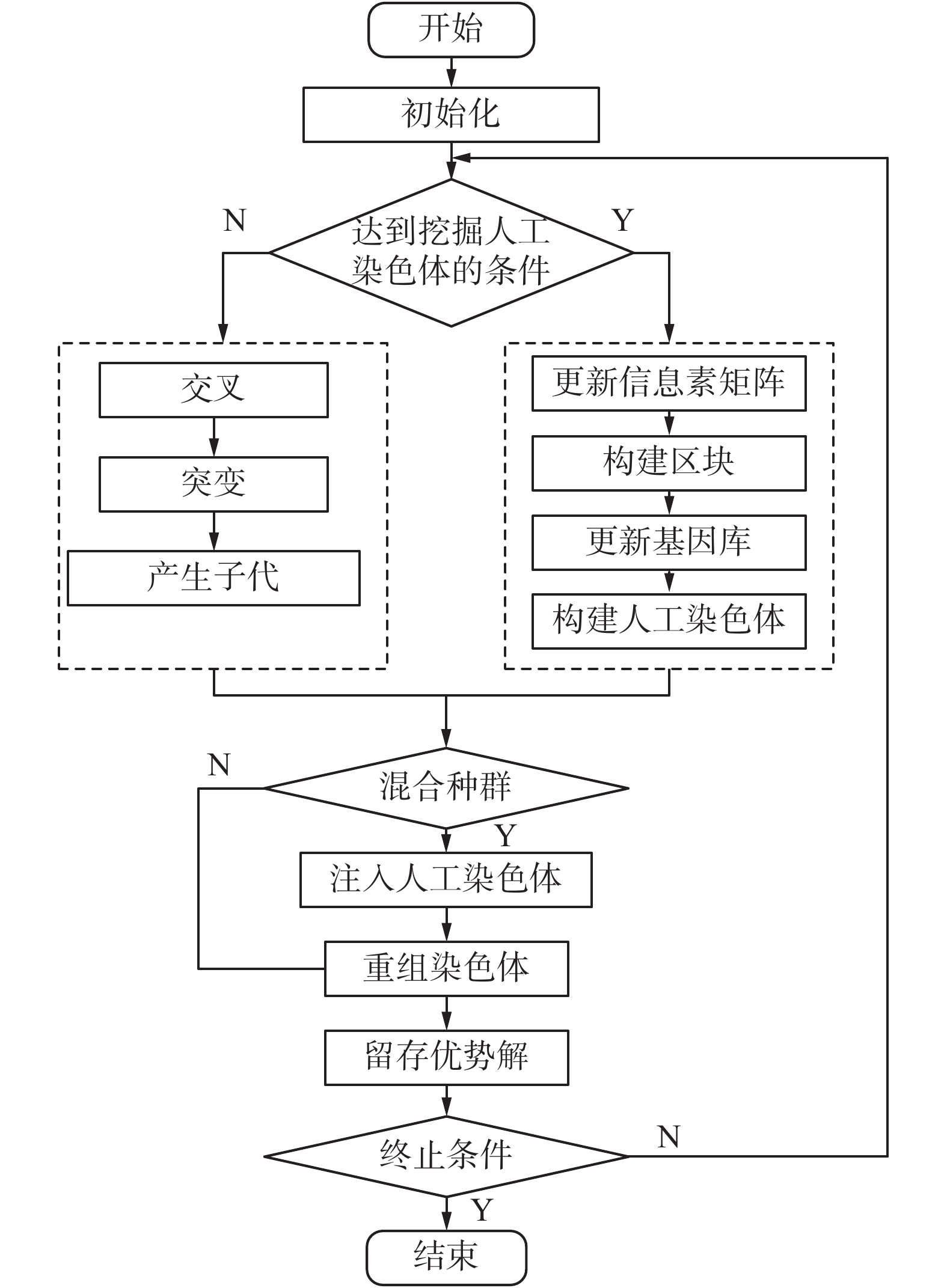Download: 图 1 p-IACGA流程 Fig. 1 Flow chart of p-IACGA
2.1 改进反向学习法初始化种群

1)反向学习法

①在 $n$ 维搜索空间中生成初始解 $X = ({x_1},{x_2}, \cdots ,$ ${x_n})$ 和反向解 $X' = ({x'_1},{x'_2}, \cdots ,{x'_n})$

②求出初始解的适应度 $f(x)$ 和其反向解的适应度 $f(x')$ ，评估两个解的适应度；

③如果 $f(x') \geqslant f(x)$ ，用 $x'$ 替换 $x$ ；否则，继续使用 $x$

2)应用改进反向学习法进行种群初始化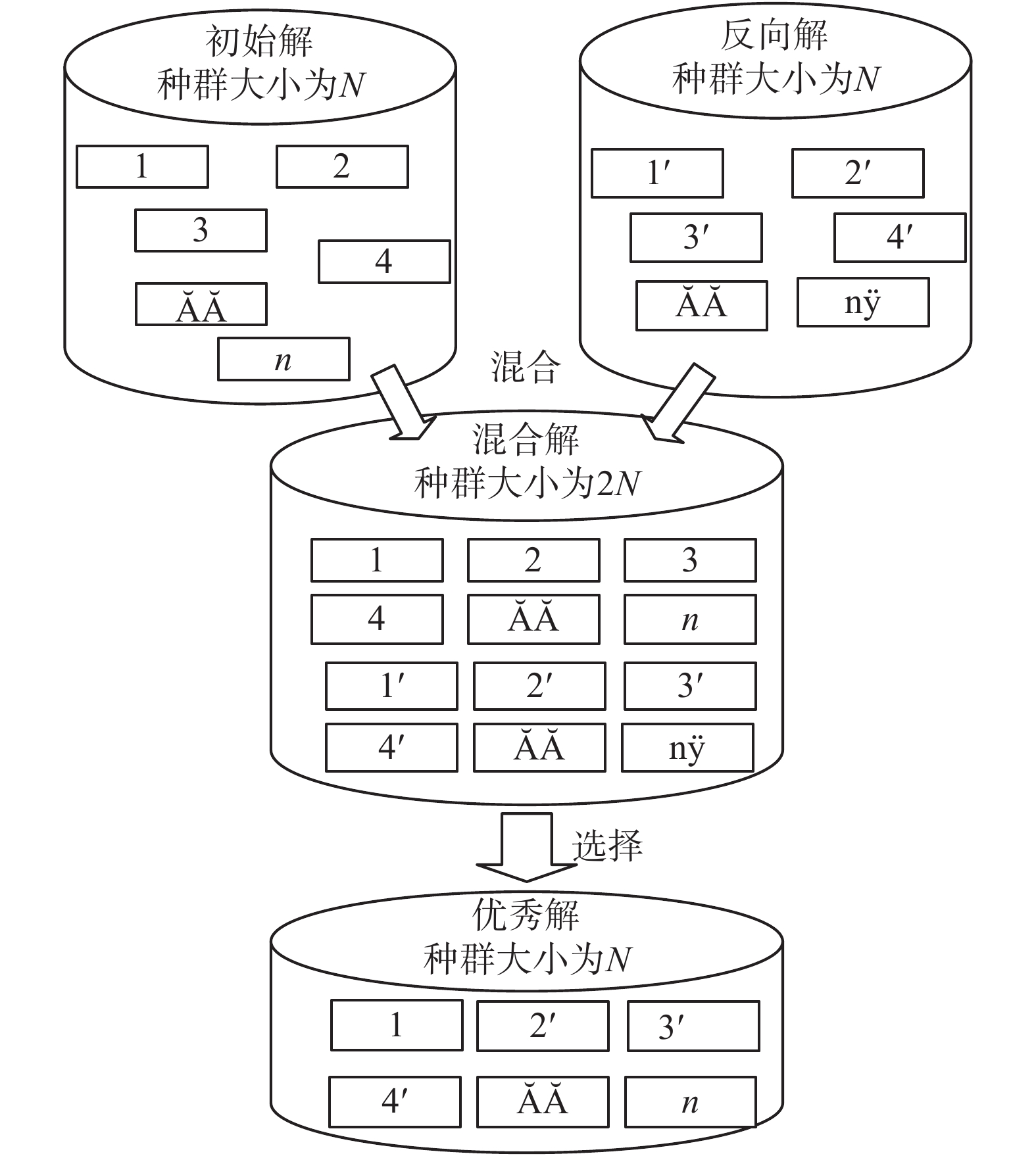Download: 图 2 改进反向学习法 Fig. 2 Improved opposition-based learning
2.2 挖掘区块

1)建立信息素矩阵

①对信息素矩阵进行初始化

 ${\tau _0} = \frac{1}{L}$ (1)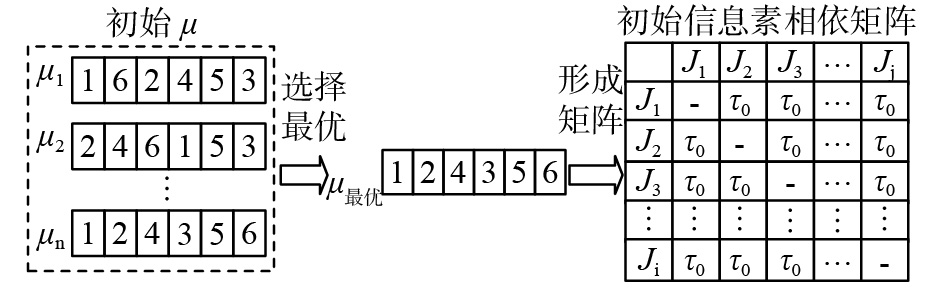Download: 图 3 初始相依信息素矩阵 Fig. 3 Initially dependent pheromone matrix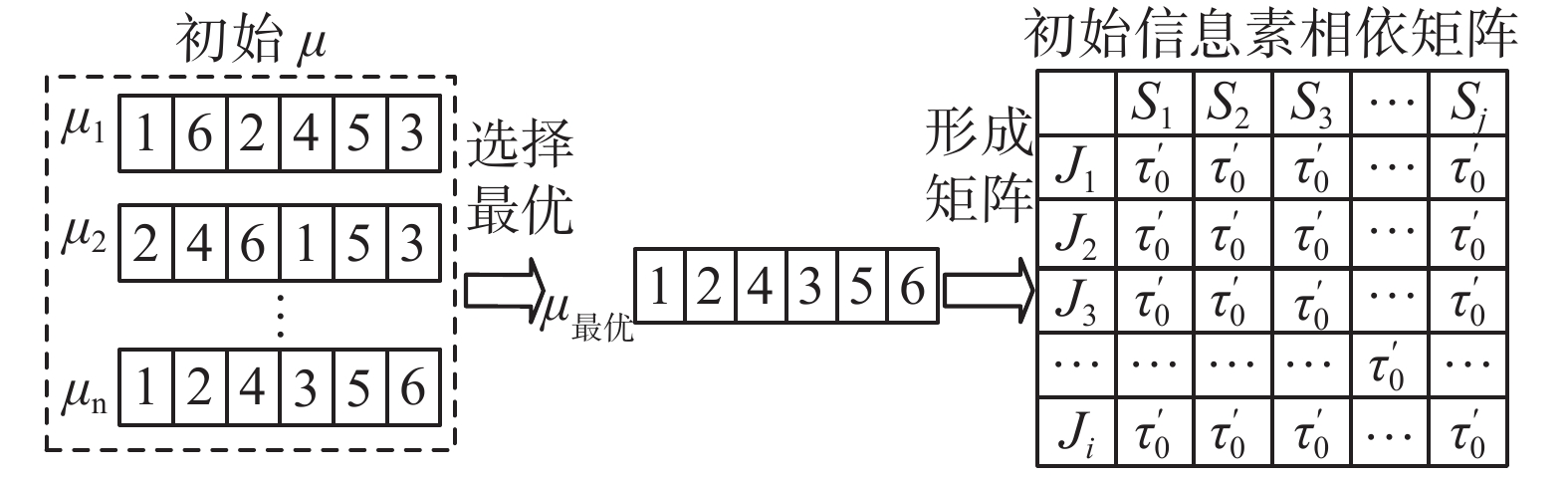Download: 图 4 初始位置信息素矩阵 Fig. 4 The initial position pheromone matrix

②更新信息素矩阵

 $\begin{array}{c} {\tau _{ij}}(t + 1) = (1 - \rho ) \times {\tau _{ij}}(t) + \rho \Delta {\tau _{ij}}(t + 1)\\ \Delta {\tau _{ij}}(t + 1) = \left\{ {\begin{array}{*{20}{l}} {1/L{\rm{ , }\quad}\text{若蚂蚁经过路径}(i,j)}\\ {0,{\rm{ }\quad}\text{其他} } \end{array}} \right. \end{array}$ (2)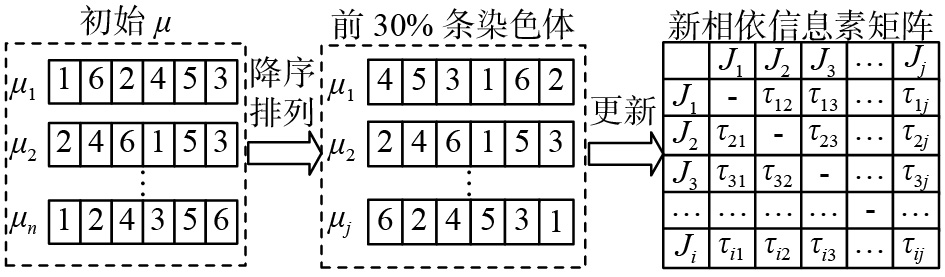Download: 图 5 更新相依信息素矩阵 Fig. 5 Update dependent pheromone matrix

 $\begin{array}{c} {\tau '_{mi}}(t + 1) = (1 - \rho ) \times {\tau '_{mi}}(t) + \rho \Delta {\tau '_{mi}}(t + 1)\\ \Delta {\tau '_{mi}}(t + 1) = \left\{ {\begin{array}{*{20}{l}} \!\!\!\!{1/L{\rm{ , }\quad}\text{若蚂蚁经过的第}m\text{个节点为节点}i}\\ \!\!\!\!{0,{\rm{ }\quad}\text{其他} } \end{array}} \right. \end{array}$ (3)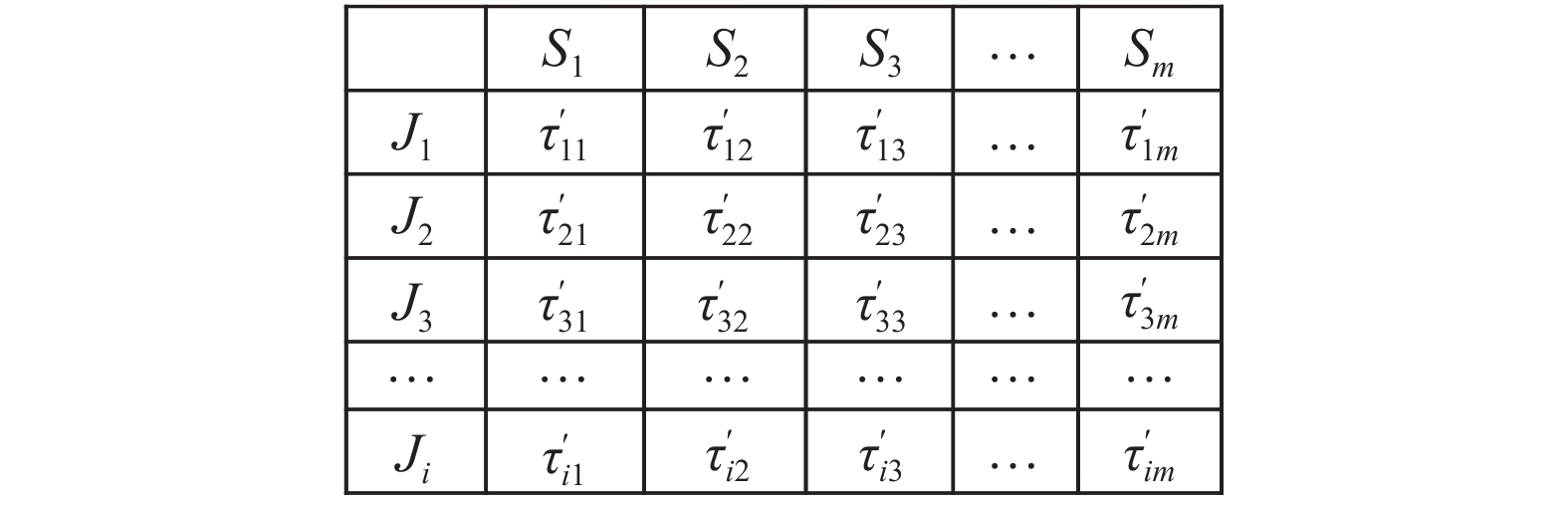Download: 图 6 更新的位置信息素矩阵 Fig. 6 Updated positional information matrix

2)构建区块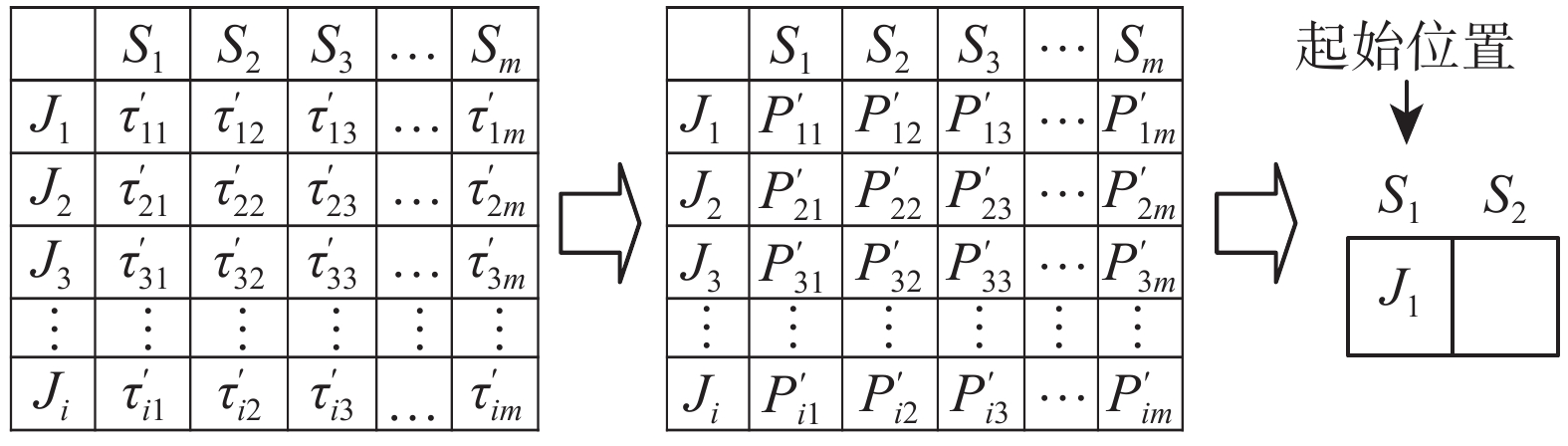Download: 图 7 起始位置工序挑选 Fig. 7 Starting position process selection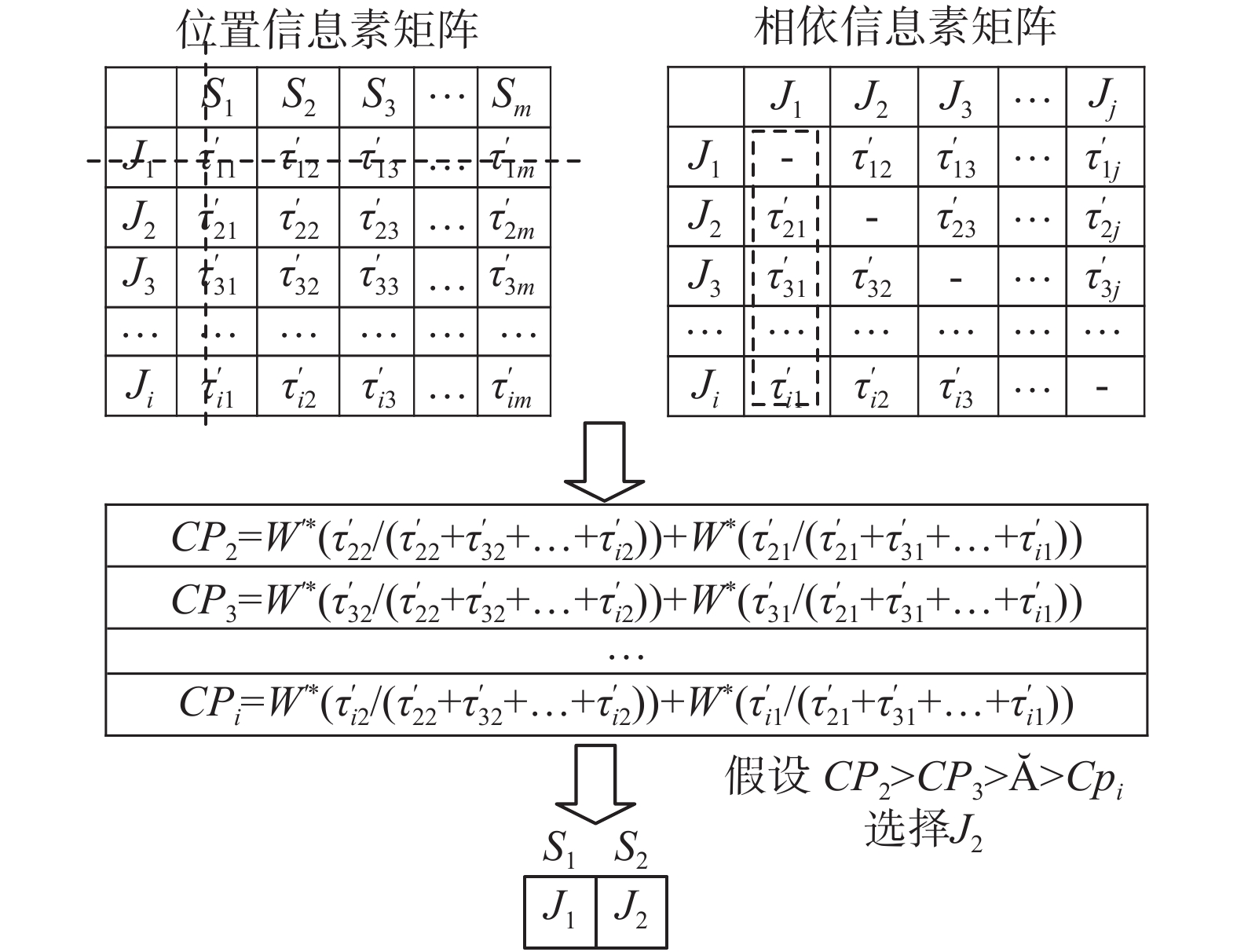Download: 图 8 区块最小长度内其他位置工件选择 Fig. 8 Workpiece selection at other positions within the block's minimum length

 ${P'_{im}} = \frac{\tau '_{mi}}{{\sum\limits_{i \in uns} {\tau '_{mi}}}}i,\quad m = 1,2, \cdots ,n$ (4)

 ${P_{ij}} = \frac{{{\tau _{ij}}}}{{\sum\limits_{i \in uns} {{\tau _{ij}}} }},\quad i,j = 1,2, \cdots ,n$ (5)

 ${{{CP}}_i} = (W \times {P_{ij}}) + (W' \times {P'_{im}}),\quad i,j,m = 1,2, \cdots ,n$ (6)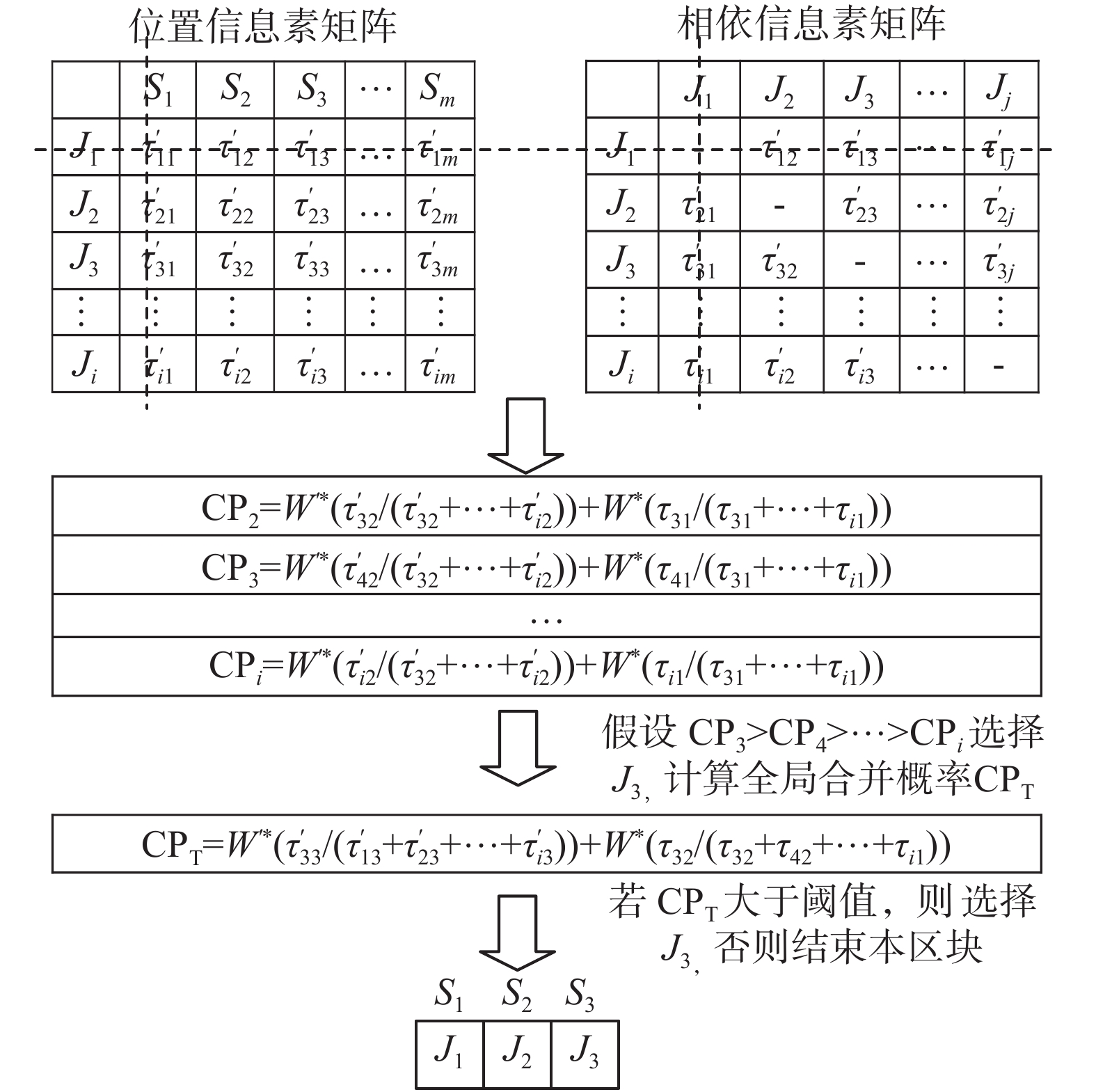Download: 图 9 区块最小长度外的工件选择 Fig. 9 Selection of workpieces outside the block minimum length

3)区块竞争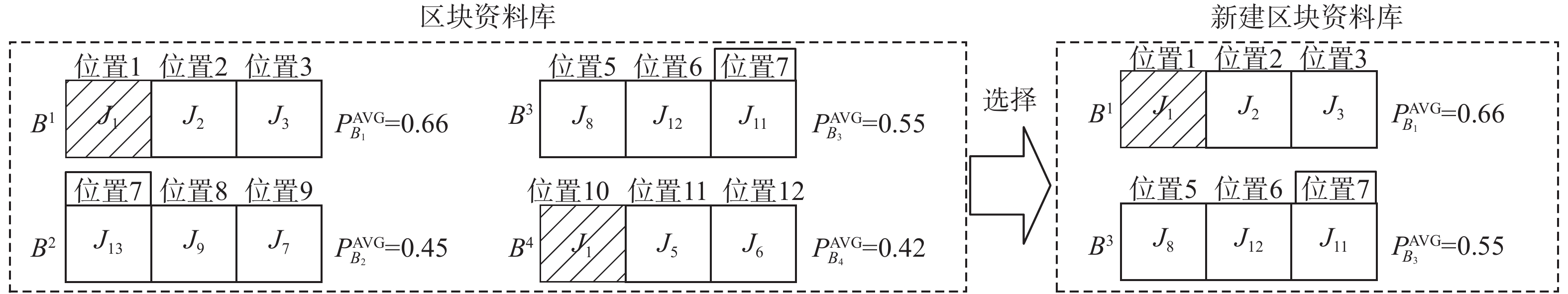Download: 图 10 区块竞争 Fig. 10 Block competition
 $P_{{B^l}}^{\rm{AVG}} = \frac{{P_{B_l^i}^{\rm{dom}} + \sum\limits_{i = 2}^n {{{{{\rm{CP}}}}_{B_l^i}}} }}{n},\quad l = 1,2, \cdots ,n$ (7)

2.3 组合染色体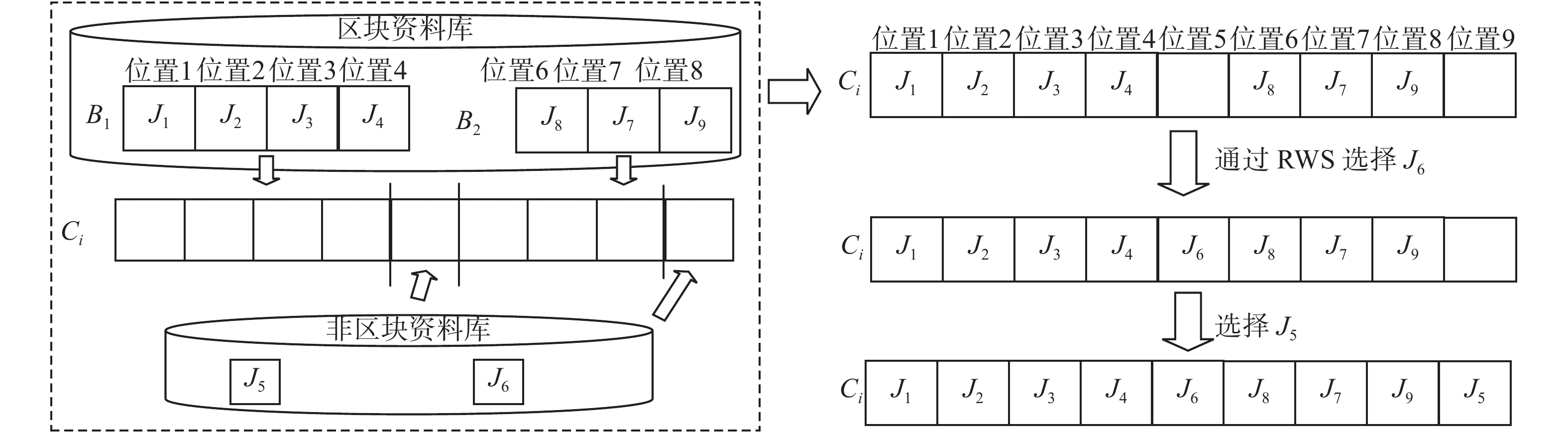Download: 图 11 染色体组合 Fig. 11 Chromosome combinations
2.4 染色体重组Download: 图 12 切割人工染色体 Fig. 12 Cutting an artificial chromosome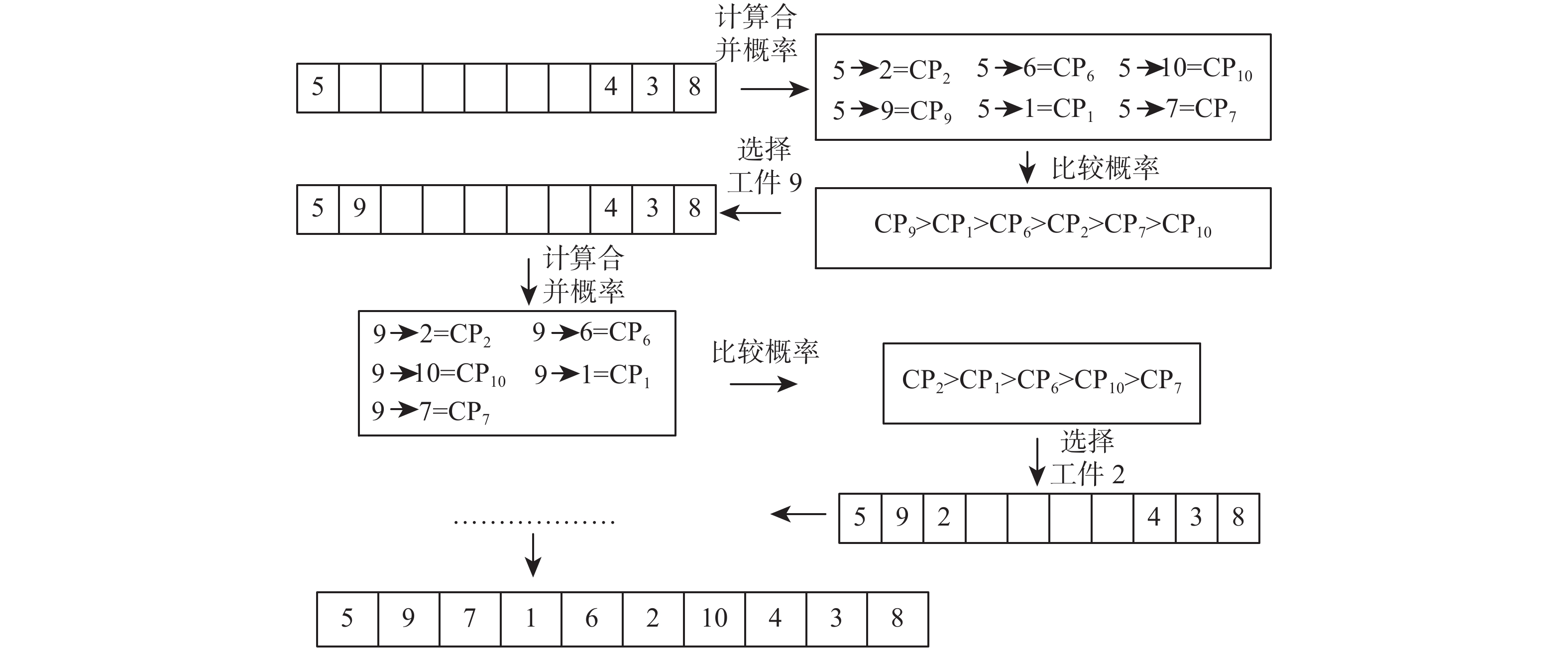Download: 图 13 人工染色体重组 Fig. 13 Artificial chromosome reorganization
2.5 留存优势解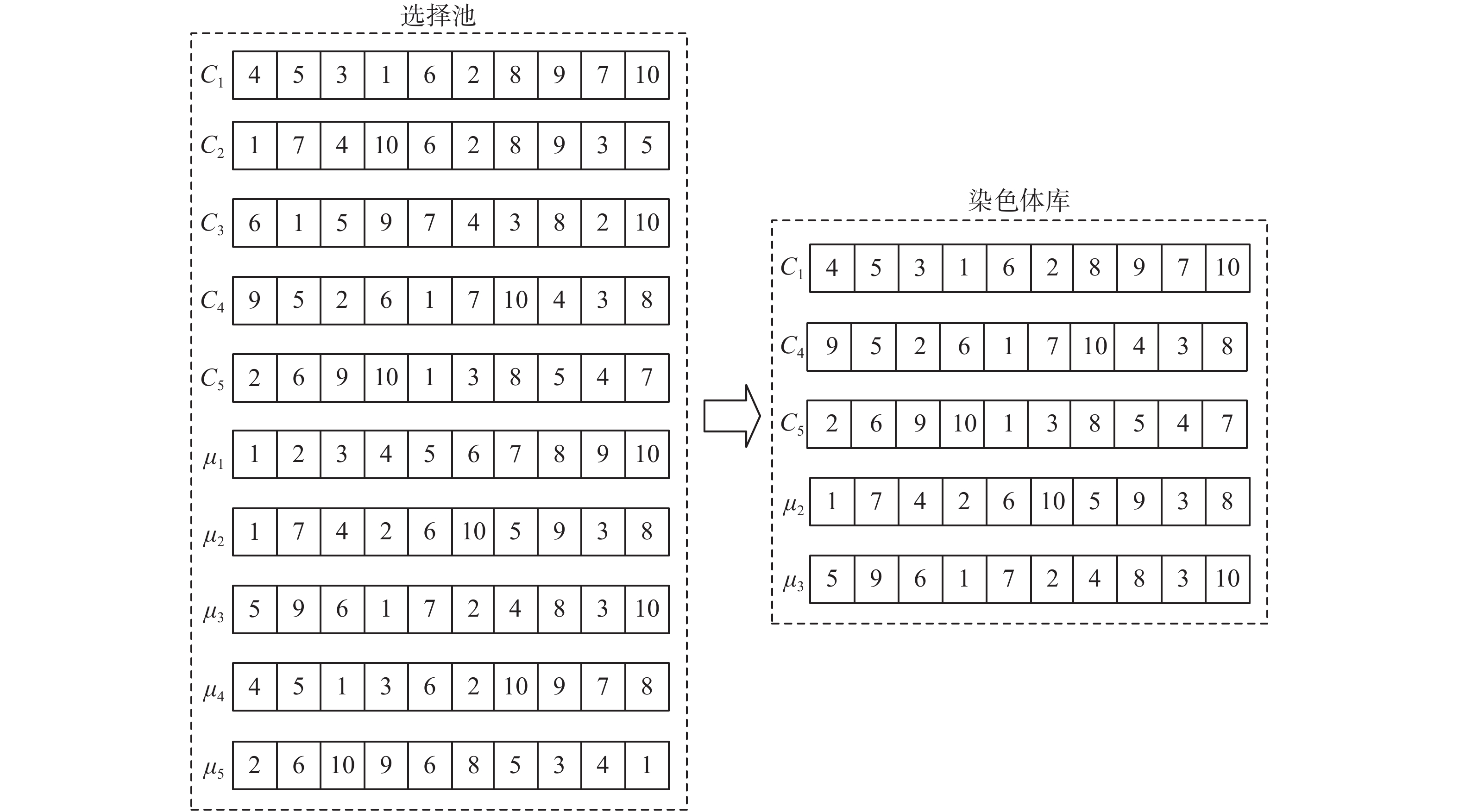Download: 图 14 留存优势解 Fig. 14 Retention of advantage solution
3 实验结果分析

 ${\rm{ER}}{_i} = \frac{{{C_{\max }} - {U_i}}}{{{U_i}}} \times 100{\text{%}}$ (8)表 1 Taillard实例测试结果比较 Tab.1 Comparison of Taillard instance test results表 2 Reeves实例测试结果比较 Tab.2 Comparison of Reeves instance test results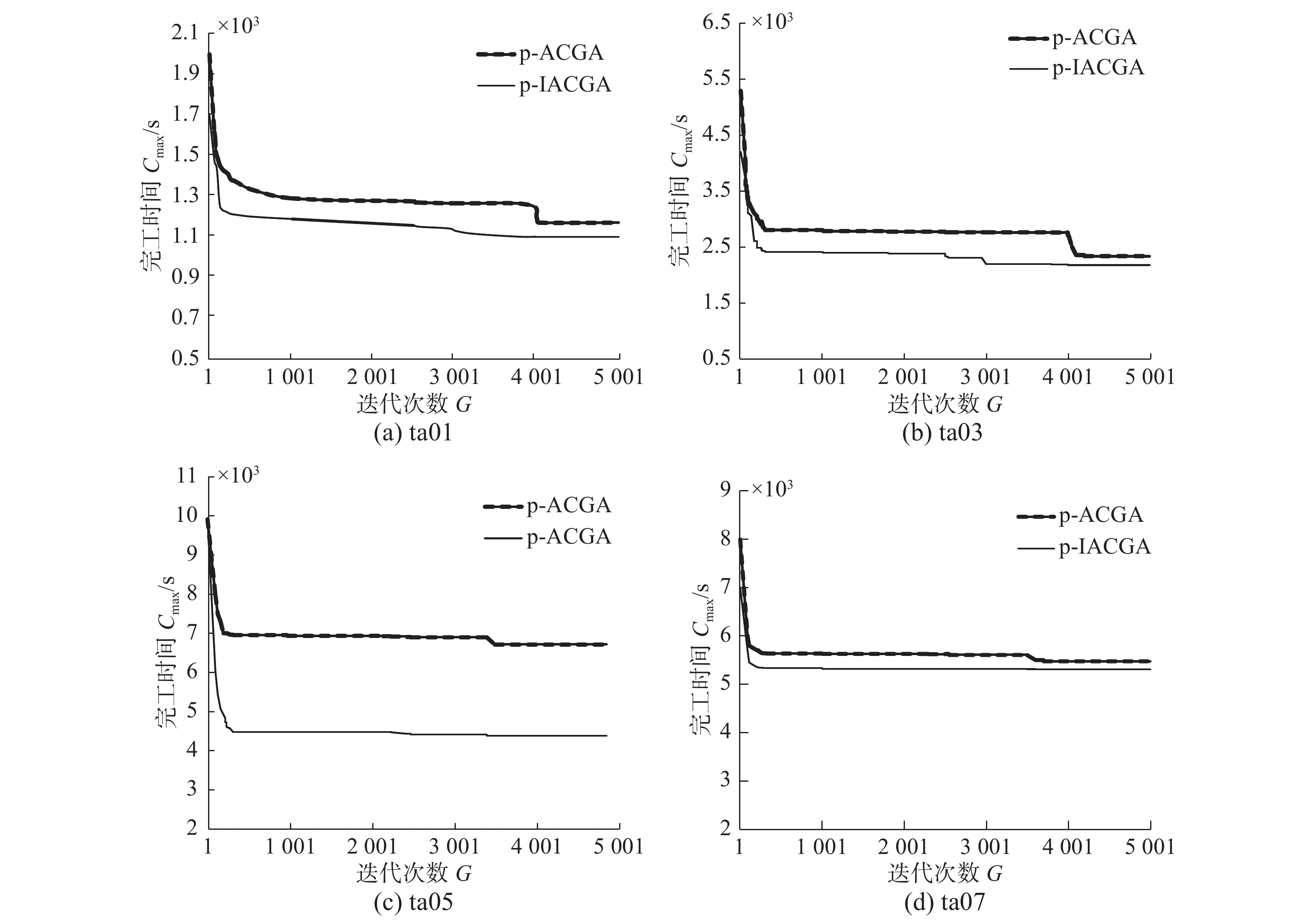Download: 图 15 算例收敛图 Fig. 15 Instance convergence diagram

4 结束语

  周明, 孙树栋. 遗传算法原理及应用[M]. 北京: 国防工业出版社, 1999: 6. (0)  夏小云, 周育人. 蚁群优化算法的理论研究进展[J]. 智能系统学报, 2016, 11(1): 27-36. XIA Xiaoyun, ZHOU Yuren. Advances in theoretical research of ant colony optimization[J]. CAAI transactions on intelligent systems, 2016, 11(1): 27-36. (0)  李金忠, 夏洁武, 曾小荟, 等. 多目标模拟退火算法及其应用研究进展[J]. 计算机工程与科学, 2013, 35(8): 77-88. LI Jinzhong, XIA Jiewu, ZENG Xiaohui, et al. Survey of multi-objective simulated annealing algorithm and its applications[J]. Computer engineering and science, 2013, 35(8): 77-88. DOI:10.3969/j.issn.1007-130X.2013.08.013 (0)  TAYEB F B S, BESSEDIK M, BENBOUZID M, et al. Research on permutation flow-shop scheduling problem based on improved genetic immune algorithm with vaccinated offspring[J]. Procedia computer science, 2017, 112: 427-436. DOI:10.1016/j.procs.2017.08.055 (0)  CHEN Rongchang, CHEN J, CHEN T S, et al. Synergy of genetic algorithm with extensive neighborhood search for the permutation flowshop scheduling problem[J]. Mathematical problems in engineering, 2017, 2017: 3630869. (0)  BESSEDIK M, TAYEB F B S, CHEURFI H, et al. An immunity-based hybrid genetic algorithms for permutation flowshop scheduling problems[J]. International journal of advanced manufacturing technology, 2016, 85(9/10/11/12): 2459-2469. (0)  GANGULY S, MUKHERJEE S, BASU D, et al. A novel strategy adaptive genetic algorithm with greedy local search for the permutation flowshop scheduling problem[M]//Swarm, Evolutionary, and Memetic Computing. Berlin, Heidelberg: Springer, 2012: 687–696. (0)  齐学梅, 王宏涛, 陈付龙, 等. 求解多目标PFSP的改进遗传算法[J]. 计算机工程与应用, 2015, 51(11): 242-247. QI Xuemei, WANG Hongtao, CHEN Fulong, et al. Improved genetic algorithm for multi-objective of PFSP[J]. Computer engineering and applications, 2015, 51(11): 242-247. DOI:10.3778/j.issn.1002-8331.1310-0197 (0)  崔琪, 吴秀丽, 余建军. 变邻域改进遗传算法求解混合流水车间调度问题[J]. 计算机集成制造系统, 2017, 23(9): 1917-1927. CUI Qi, WU Xiuli, YU Jianjun. Improved genetic algorithm variable neighborhood search for solving hybrid flow shop scheduling problem[J]. Computer integrated manufacturing systems, 2017, 23(9): 1917-1927. (0)  CHANG P C, HUANG W H, WU J L, et al. A block mining and re-combination enhanced genetic algorithm for the permutation flowshop scheduling problem[J]. International journal of production economics, 2013, 141(1): 45-55. DOI:10.1016/j.ijpe.2012.06.007 (0)  JIN Jian. A hybrid discrete biogeography-based optimization for the permutation flow shop scheduling problem[J]. International journal of production research, 2016, 54(16): 4805-4814. DOI:10.1080/00207543.2015.1094584 (0)  DORIGO M, GAMBARDELLA L M. Ant colony system: a cooperative learning approach to the traveling salesman problem[J]. IEEE transactions on evolutionary computation, 1997, 1(1): 53-66. DOI:10.1109/4235.585892 (0)  陈慧芬. 基于链结学习的子群体进化算法求解多目标调度问题[D]. 天津: 天津理工大学, 2017. CHEN Huifen. Sub-population evolutionary algorithm based on linkage learning for multi-objective scheduling problem[D]. Tianjin: Tianjin University of Technology, 2017. (0)  裴小兵, 陈慧芬, 张百栈, 等. 改善式BVEDA求解多目标调度问题[J]. 山东大学学报(工学版), 2017, 47(4): 25-30. PEI Xiaobing, CHEN Huifen, ZHANG Baizhan, et al. Improved bi-variables estimation of distribution algorithms for multi-objective permutation flow shop scheduling problem[J]. Journal of Shandong University (engineering science), 2017, 47(4): 25-30. (0)  裴小兵, 赵衡. 基于二元分布估计算法的置换流水车间调度方法[J]. 中国机械工程, 2017, 28(22): 2752-2759. PEI Xiaobing, ZHAO Heng. Permutation flow shop scheduling problem based on hybrid binary distribution estimation algorithm[J]. China mechanical engineering, 2017, 28(22): 2752-2759. DOI:10.3969/j.issn.1004-132X.2017.22.016 (0)  张敏, 汪洋, 方侃. 基于改进区块进化算法求解置换流水车间问题[J]. 计算机集成制造系统, 2018, 24(5): 1207-1216. ZHANG Min, WANG Yang, FANG Kan. Improved block-based evolutionary algorithm for solving permutation flowshop scheduling problem[J]. Computer integrated manufacturing systems, 2018, 24(5): 1207-1216. (0)  CHANG P C, CHEN Menghui. A block based estimation of distribution algorithm using bivariate model for scheduling problems[J]. Soft computing, 2014, 18(6): 1177-1188. DOI:10.1007/s00500-013-1136-1 (0)  MILLER B L, GOLDBERG D E. Genetic algorithms, tournament selection, and the effects of noise[J]. Complex systems, 1995, 9(3): 193-212. (0)  CHANG P C, CHEN S H, FAN C Y, et al. Generating artificial chromosomes with probability control in genetic algorithm for machine scheduling problems[J]. Annals of operations research, 2010, 180(1): 197-211. DOI:10.1007/s10479-008-0489-9 (0)  HSU C Y, CHANG P C, CHEN M H. A linkage mining in block-based evolutionary algorithm for permutation flowshop scheduling problem[J]. Computers & industrial engineering, 2015, 83: 159-171. (0)